SSAT Middle Level Math : Fractions

Example Questions

Example Question #841 : Fractions

Express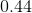as a fraction in simplest form.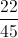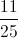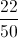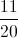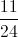Explanation:is forty-four one-hundredths, so write this as a fraction and reduce: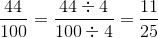Example Question #841 : Fractions

Write as a decimal: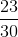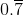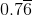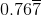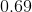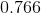Explanation:

Divideby: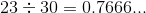The 6 repeats infinitely, so this can be written asExample Question #843 : Fractions

What decimal is equivalent to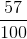?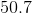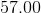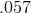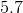Explanation:is fifty-seven hundredths.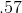is fifty-seven hundredths. When we say a decimal, we say the number and add the place-value of the last digit.

Example Question #844 : Fractions

What decimal is equivalent to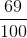?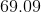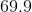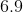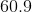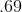Explanation:is sixty-nine hundredths.is sixty-nine hundredths. When we say a decimal, we say the number and add the place-value of the last digit.

Example Question #101 : Use Decimal Notation For Fractions With Denominators 10 Or 100: Ccss.Math.Content.4.Nf.C.6

What decimal is equivalent to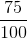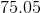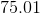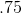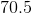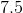Explanation:is seventy-five hundredths.is seventy-five hundredths. When we say a decimal, we say the number and add the place-value of the last digit.

Example Question #101 : Use Decimal Notation For Fractions With Denominators 10 Or 100: Ccss.Math.Content.4.Nf.C.6

What decimal is equivalent to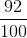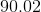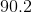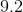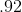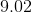Explanation:is ninety-two hundredths.is ninety-two hundredths. When we say a decimal, we say the number and add the place-value of the last digit.

Example Question #845 : Fractions

What decimal is equivalent to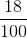?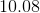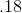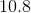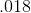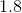Explanation:is eighteen hundredths.is eighteen hundredths. When we say a decimal, we say the number and add the place-value of the last digit.

Example Question #846 : Fractions

What decimal is equivalent to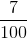?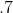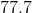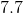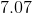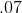Explanation:is seven hundredths.is seven hundredths. When we say a decimal, we say the number and add the place-value of the last digit.

Example Question #21 : Understand Decimal Notation For Fractions, And Compare Decimal Fractions

Select the decimal that is equivalent to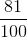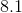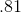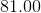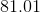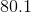Explanation:is eighty-one hundredths.is eighty-one hundredths. When we say a decimal, we say the number and add the place-value of the last digit.

Example Question #1 : Use Decimal Notation For Fractions With Denominators 10 Or 100: Ccss.Math.Content.4.Nf.C.6

Select the decimal that is equivalent to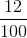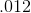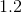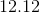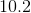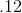is twelve hundredths.is twelve hundredths. When we say a decimal, we say the number and add the place-value of the last digit.